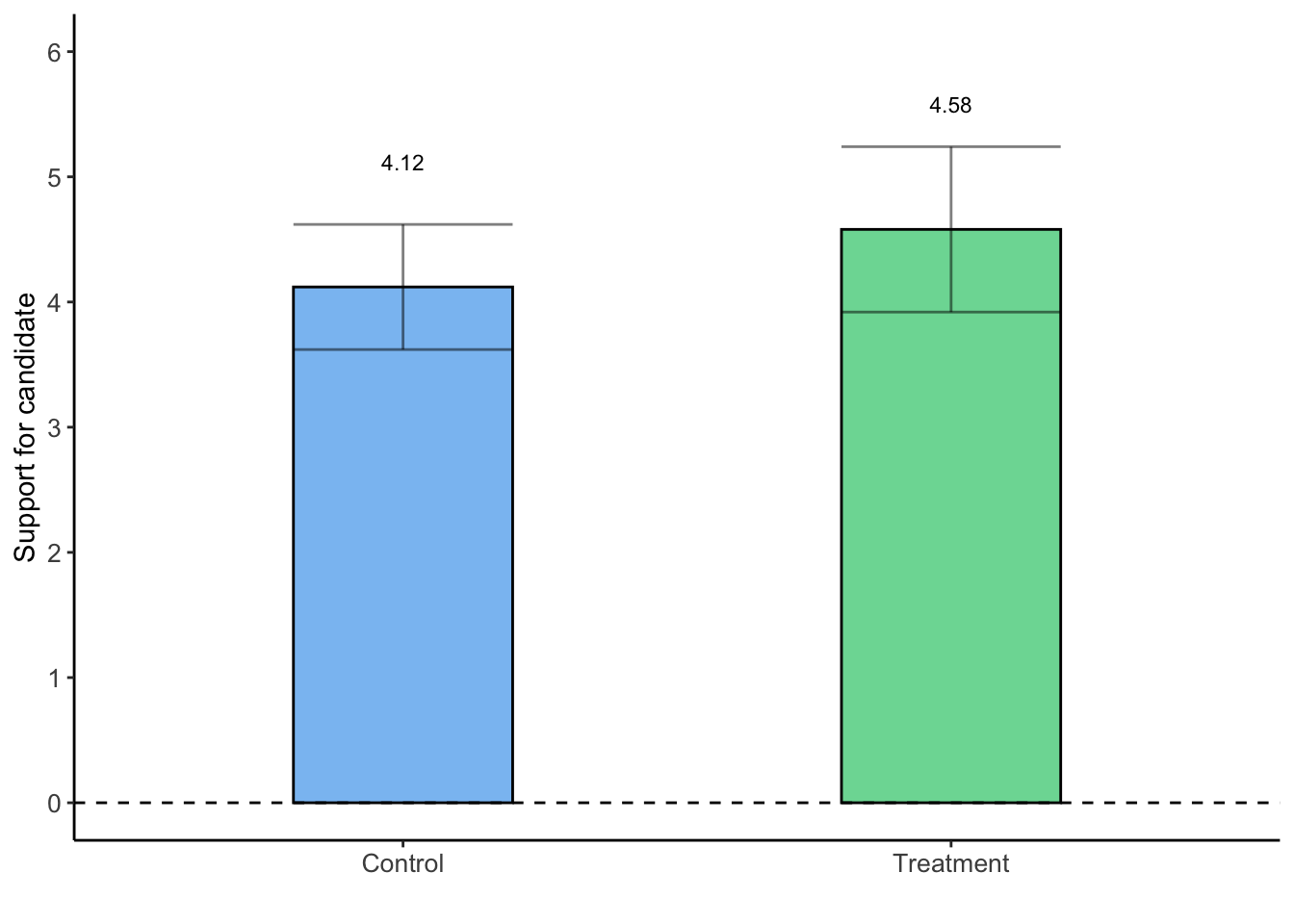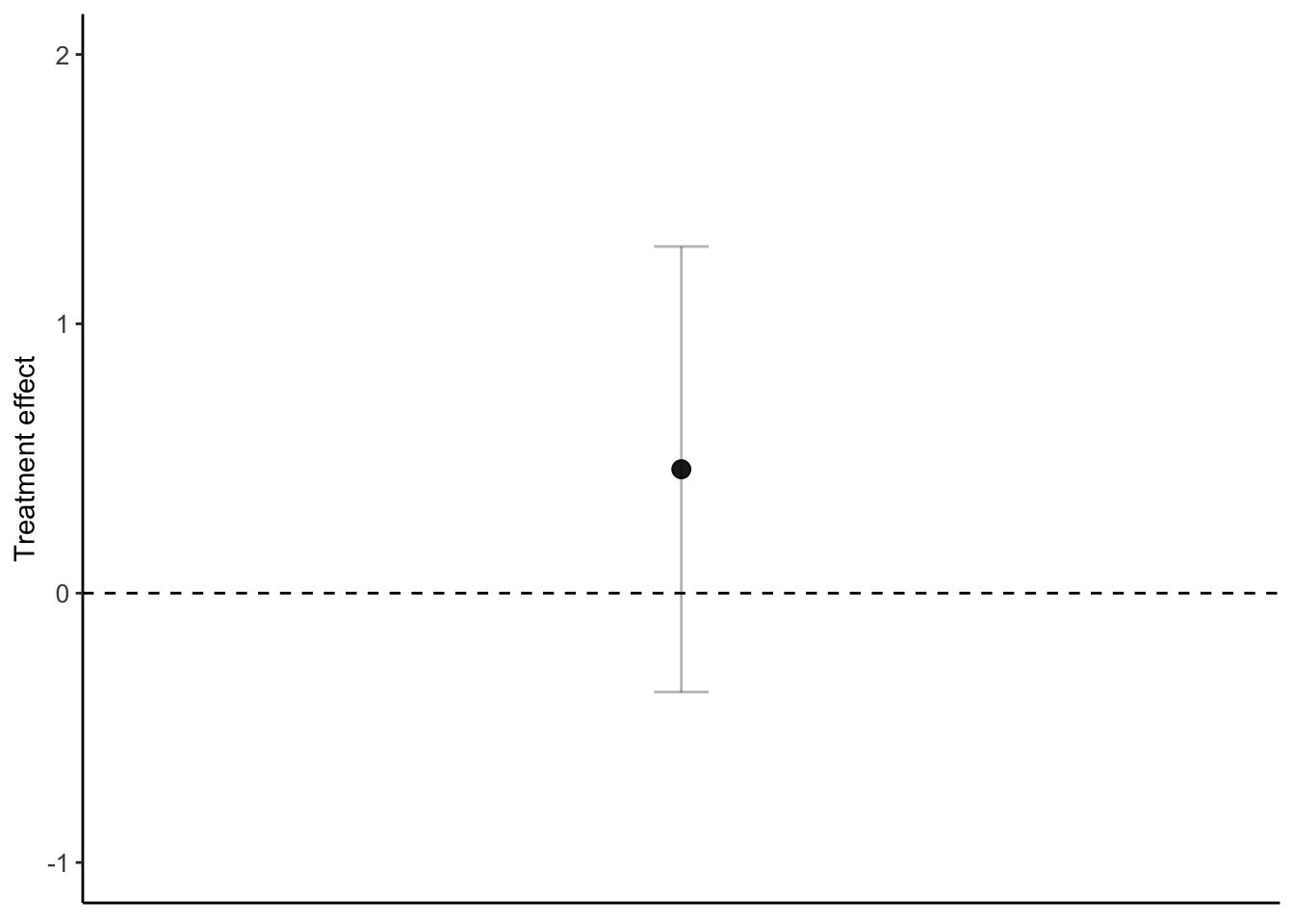## The bootstrap

A way of estimating statistical parameters (e.g. the population mean and its confidence interval) from the sample by means of resampling with replacement.

Example of the bootstrap procedure. Finding a bootstrapped standard error of a difference-in-means estimate of a randomized experiment.

1. Randomly sample with replacement n times.
2. Calculate our would-be estimate using this bootstrap sample. In this case, this means we will calculate the difference-in-means from our new bootstrapped sample. We will then store this bootstrapped estimate of the difference-in-means.
3. Repeat steps 1 and 2 many times until we have a large sample of stored bootstrapped estimates.
4. Using the resulting collection of bootstrap estimates, calculate the standard deviation of the bootstrap distribution of our estimator.

First, let’s calculate the difference-in-means and standard errors analytically.

``````# Load PS4 csv

# Obtain the differences in means estimate of the effect of Z on Y.
dim <- mean(df1[df1\$Z == 1, "Y"]) - mean(df1[df1\$Z == 0, "Y"])

# # Create a dataframe showing us groupmeans and standard errors
groupmeans <- df1 %>%
group_by(Z) %>%
summarise(groupmean = mean(Y, na.rm = TRUE), size = n(), var = var(Y))

# Calculate standard errors
groupmeans\$se <- sqrt(groupmeans\$var/groupmeans\$size)
groupmeans\$se <- sqrt(groupmeans\$var/groupmeans\$size)

# Calculate the analytic standard error of your estimate
se = with(groupmeans,
sqrt(groupmeans[Z == 1, "se"]^2 + groupmeans[Z == 0, "se"]^2))
se_analytic = se[1, 1]``````

Now let’s plot this thing to make sure it looks reasonable.

``````# Create chart
ggplot(groupmeans, aes(factor(Z), groupmean, fill = factor(Z))) +
geom_col(position = "dodge",
color = "black", alpha = 0.75,
width = 0.4) +
scale_fill_manual(values = c("steelblue2", "seagreen3"), guide = FALSE) +
geom_errorbar(aes(ymin = groupmean - 1.96*se, ymax = groupmean + 1.96*se),
position = position_dodge(),
color="black", alpha = 0.5,
size=0.5,
width = 0.4) +
geom_text(aes(label = round(groupmean, 2)), nudge_y = 1, size = 3) +
geom_hline(yintercept = 0, linetype = "dashed") +
xlab("Treatment") +
ylab("Support for candidate") +
scale_x_discrete("", labels = c("0" = "Control", "1" = "Treatment")) +
scale_y_continuous(limits = c(0, 6), breaks=seq(0,6, 1)) +
theme_classic() +
theme(plot.title = element_text(hjust = 0.5)) +
theme(axis.text=element_text(size = 10)) +
theme(legend.position = "none")``````Now let’s estimate our standard errors using the bootstrap

``````# Set the number of times we will sample with replacement
sims <- 10000

# Create an empty vector to store our bootstrap estimates
boot_ests <- rep(NA, sims)

# Run a loop that follows the bootstrap procedure
for (i in 1:sims) {

# Sample WITH REPLACEMENT 100 times
sample_indicies = sample(1:nrow(df1), size = nrow(df1), replace = TRUE)

# Create new dataframe of samples
boot_df = df1[sample_indicies, , drop = FALSE]

# Calculate our estimate (the DIM) from this sample
boot_ests[i] <- with(boot_df, mean(boot_df[Z == 1, "Y"]) -
mean(boot_df[Z == 0, "Y"]))
}

# Calculate the standard error of our bootstrap estimate
se_boot <- sd(boot_ests)``````

Let’s check to see if our analytic standard errors and bootstrapped standard errors are similar.

``````# Confirm that analytic estimates and manually calculated estimates are similar
if(all.equal(se_boot, se_analytic, tolerance = 0.01))
{print("Estimates are nearly identical")}``````
``##  "Estimates are nearly identical"``

## Regression

### Bivariate regression example

``````# Run a bivariate regression in R
dim = lm(Y ~ Z, data = df1)
summary(dim)``````
``````##
## Call:
## lm(formula = Y ~ Z, data = df1)
##
## Residuals:
##    Min     1Q Median     3Q    Max
##  -4.58  -1.58  -0.12   0.88   4.88
##
## Coefficients:
##             Estimate Std. Error t value Pr(>|t|)
## (Intercept)   4.1200     0.2988  13.789   <2e-16 ***
## Z             0.4600     0.4226   1.089    0.279
## ---
## Signif. codes:  0 '***' 0.001 '**' 0.01 '*' 0.05 '.' 0.1 ' ' 1
##
## Residual standard error: 2.113 on 98 degrees of freedom
## Multiple R-squared:  0.01195,    Adjusted R-squared:  0.001866
## F-statistic: 1.185 on 1 and 98 DF,  p-value: 0.279``````
``````# Show that alpha and beta are equal to what they should be
alpha = mean(df1\$Y) - cov(df1\$Z, df1\$Y)/var(df1\$Z) * mean(df1\$Z)
beta = cov(df1\$Z, df1\$Y)/var(df1\$Z)

print(alpha)``````
``##  4.12``
``print(beta)``
``##  0.46``

Now lets output a regression table

``````# Output regression table using stargazer
stargazer(dim,
dep.var.labels = "Support for candidate",
covariate.labels = c("Treatment", "Constant"),
title = "Average tresatment effect: treatment on candidate support ",
align = TRUE,
omit.stat = c("f", "rsq", "ser"),
type = "html")``````
 Dependent variable: Support for candidate Treatment 0.460 (0.423) Constant 4.120*** (0.299) Observations 100 Adjusted R2 0.002 Note: p<0.1; p<0.05; p<0.01
``````# Side note: to calcualte robust standard errors in R
# and integrate with stargazer
# Calculate robust standard errors
cov = vcovHC(dim, type = "HC")
robust_se = sqrt(diag(cov))

stargazer(dim,
se = list(robust_se),
dep.var.labels = "Support for candidate",
covariate.labels = c("Treatment", "Constant"),
title = "Average tresatment effect: treatment on candidate support ",
align = TRUE,
omit.stat = c("f", "rsq", "ser"),
type = "html")``````
 Dependent variable: Support for candidate Treatment 0.460 (0.418) Constant 4.120*** (0.252) Observations 100 Adjusted R2 0.002 Note: p<0.1; p<0.05; p<0.01

### Bootstrapping regression standard errors

``````################################################################################
# Bootstrap regression standard errors
###############################################################################
# Run OLS model
dim = lm(Y ~ Z, data = df1)

# Extract treatment coefficient estimate
dim_coef <- dim\$coefficients

# Calculate bootstrapped standard errors
set.seed(5)
sims = 10000

treatment_boot = rep(NA, sims)

for(i in 1:sims){
# Sample with replacement
boot = df1[sample(nrow(df1), replace = TRUE), ]

# Run OLS model
fit_boot = lm(Y ~ Z, data = boot)

# Store coefficients
treatment_boot[i] = fit_boot\$coefficients
}

# Calculate standard errors
se_boot_reg = sd(treatment_boot)

# Calculate confidence interval
ci_upper = dim_coef + 1.96 * se_boot_reg
ci_lower = dim_coef - 1.96 * se_boot_reg

# Store information in dataframe
dim_df = data.frame(dim_coef, se_boot_reg, ci_upper, ci_lower)``````

Lets check to see if our bootstrapped standard error from the regression is similar to what we calculated before using the analytic estimate and our bootstrapped difference-in-means.

``print(se_boot_reg)``
``##  0.4220079``

Create a coefficient plot showing the treatment effect and a confidence interval derived from your bootstrapped SE.

``````# Create treatment indicator variable in dataframe
dim_df\$treatment = 1

# Create coefficient plot of treatment effects
ggplot(dim_df, aes(treatment, dim_coef)) +
geom_point(size = 3, alpha = 0.9)+
geom_errorbar(aes(ymin = ci_lower, ymax = ci_upper),
position = position_dodge(),
color="black", alpha = 0.3,
size=0.5,
width = 0.1) +
scale_y_continuous(limits = c(-1, 2)) +
scale_x_continuous(limits = c(0, 2)) +
geom_hline(yintercept = 0, linetype = "dashed") +
ylab("Treatment effect") +
theme_classic() +
theme(plot.title = element_text(hjust = 0.5)) +
theme(axis.text=element_text(size = 10)) +
theme(axis.title.x=element_blank()) +
theme(axis.text.x=element_blank()) +
theme(axis.ticks.x=element_blank())``````